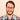# Why you should be using it

The enumerate built-in function it's a great way to iterate over something. Your code will be more readable and you have some flexibility when building a loop. You can use enumerate in any sequence, iterator or another object that supports iteration.

Instead of writing a loop like this `for i in range(len(foo))` to get the index of `foo` a better way would be to use the enumerate, because this function returns the index and value of `foo`.

# Using Enumerate in a Loop

Using enumerate in a loop is quite easy. You can either pass one or two variables to the for loop and then wrap the thing you want to loop over with the `enumerate()` function.

The difference between passing one or two variables is the result returned to you. If you pass one variable, you'll get a tuple. If you pass two variables, you'll get an `int` containing the index number and the value.

So a loop can be written with a single variable like this: `for i in enumerate(foo)`

You would get the following tuple: `(1, 'bar')`

Or you can pass two variables into the loop like this: `for index, value in enumerate(foo)`

You will get the following two values: `1 'bar'`

# Basic Example

Now that you know, what you will get, if you pass one or two variables to a for loop, let's see an example where `enumerate()` is used.

Let's imagine, you created a list containing the names of your neighbours. The list looks like this:

`names = ["John", "Leah", "Bob", "Thomas", "John", "Bob", "Bob"]`

Next, you want to know the index of every name, so you can do something with it. If you pass only one variable to the loop you will get this tuple:

```python```1for name in enumerate(names):2    print(name)3
4(0, 'John')5(1, 'Leah')6(2, 'Bob')7(3, 'Thomas')8(4, 'John')9(5, 'Bob')10(6, 'Bob')``````

But if you use two variables you will get the following result:

```python```1for index, name in enumerate(names):2    print(index, name)3
40 John51 Leah62 Bob73 Thomas84 John95 Bob106 Bob``````

As you can see, you could use two variables, if you want more flexibility and make your code more readable.

# Example: Hamming difference between two DNA strands

Disclaimer: This part contains my solution of the hamming problem found on exercism.io

This example is taken from one of the many exercises, that you can get from exercism.io. I recommend you to check it, as the challenges are pretty awesome and fun to complete!

The Hamming distance is a measure of similarity between two strings of equal length and it measures the minimum number of substitutions required to change one string into the other.

In the exercism challenge we have to calculate the Hamming difference between two DNA strands.

Example:

```git```1GAGCCTACTAACGGGAT2CATCGTAATGACGGCCT3^ ^ ^  ^ ^    ^^4
5The Hamming distance between these two DNA strands is 7.``````

This is a perfect exercise for us to use our knowledge of the enumerate built-in function and solve this problem.

Exercism.io provides us with a few test cases to check if our solution is adequate. Some of the test cases look like this:

```python```1def test_large_distance(self):2    self.assertEqual(hamming.distance("GATACA", "GCATAA"), 4)3
4def test_large_distance_in_off_by_one_strand(self):5    self.assertEqual(hamming.distance("GGACGGATTCTG", "AGGACGGATTCT"), 9)6
7def test_empty_strands(self):8    self.assertEqual(hamming.distance("", ""), 0)9
10def test_disallow_first_strand_longer(self):11    with self.assertRaises(ValueError):12        hamming.distance("AATG", "AAA")``````

## Solving the problem

To solve this issue we will need:

• A place to add up all the different occurrences between two strings
• A test to see if the two strings are the same length
• A loop to test the two indexes together

Now that we have all the tools needed, building the function is pretty easy.

`python`11   def distance(first_strand, second_strand):22       total = 033       if len(first_strand) != len(second_strand):44           raise ValueError55       for index, value in enumerate(first_strand):66           if first_strand[index] != second_strand[index]:77               total += 188       return total``

(line 3) Due to the hamming constraint, we first check if both strings are the same length.

(line 5) I've opted to use two variables in the for loop, because it makes the code more readable. Since all we need is the index number, we could just use the first value of the tuple.

0 Like 0 Comment

### You might also like these